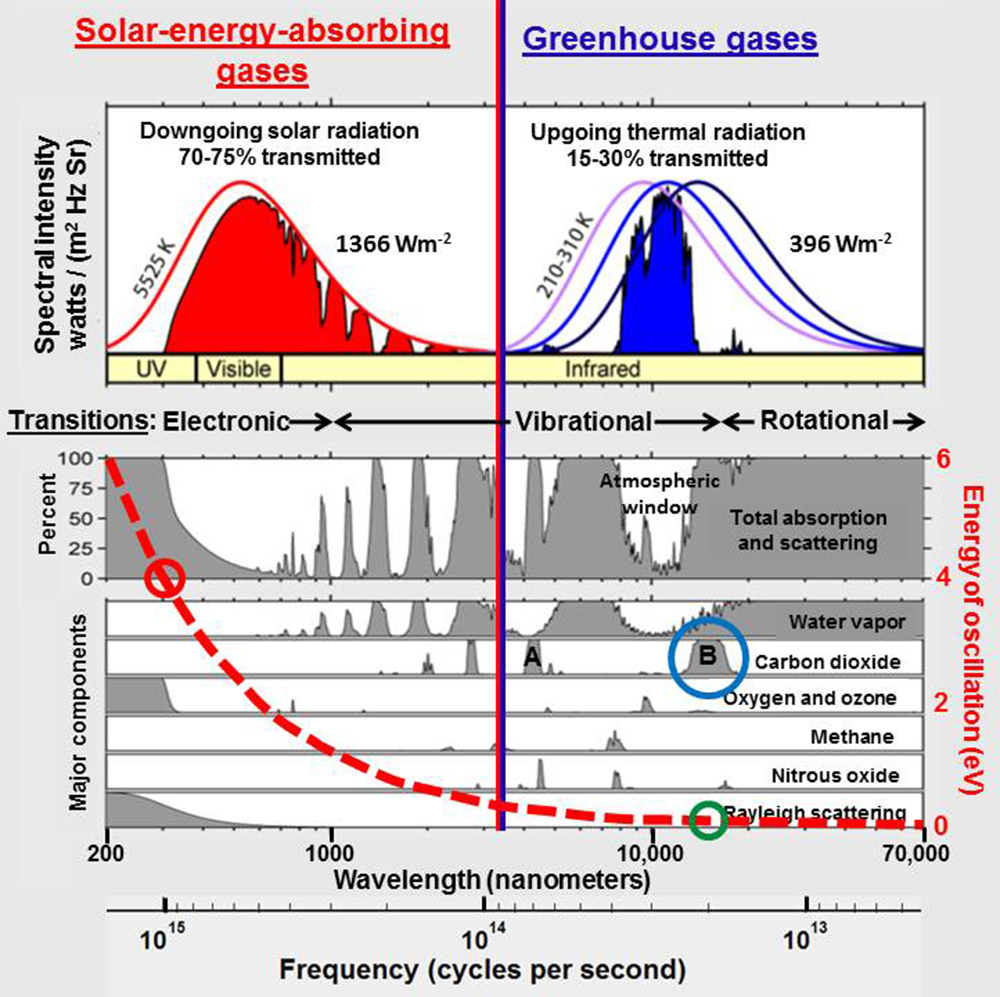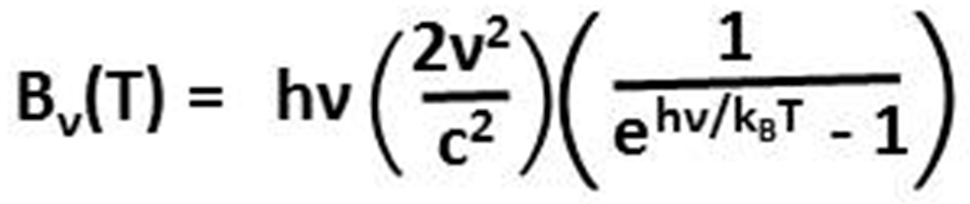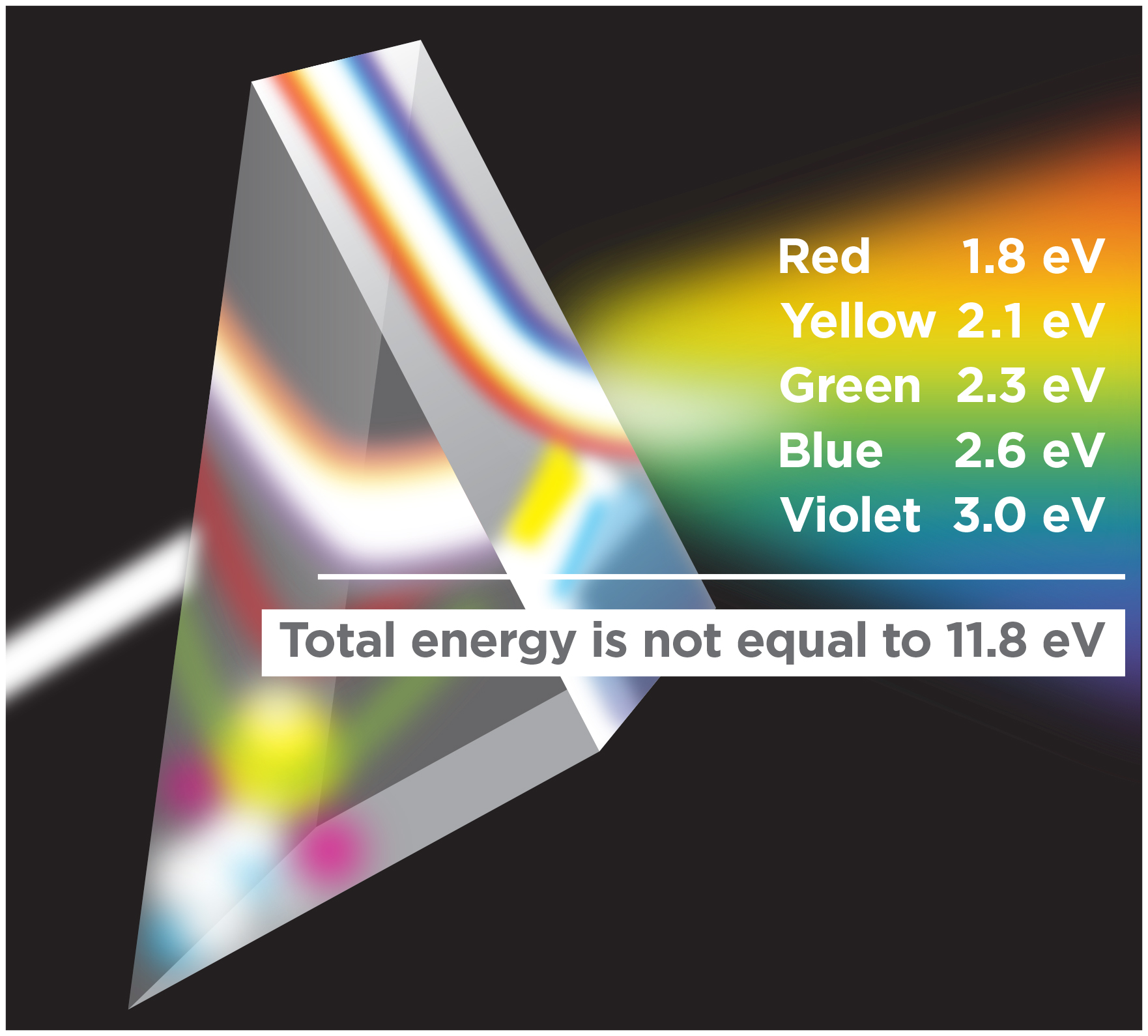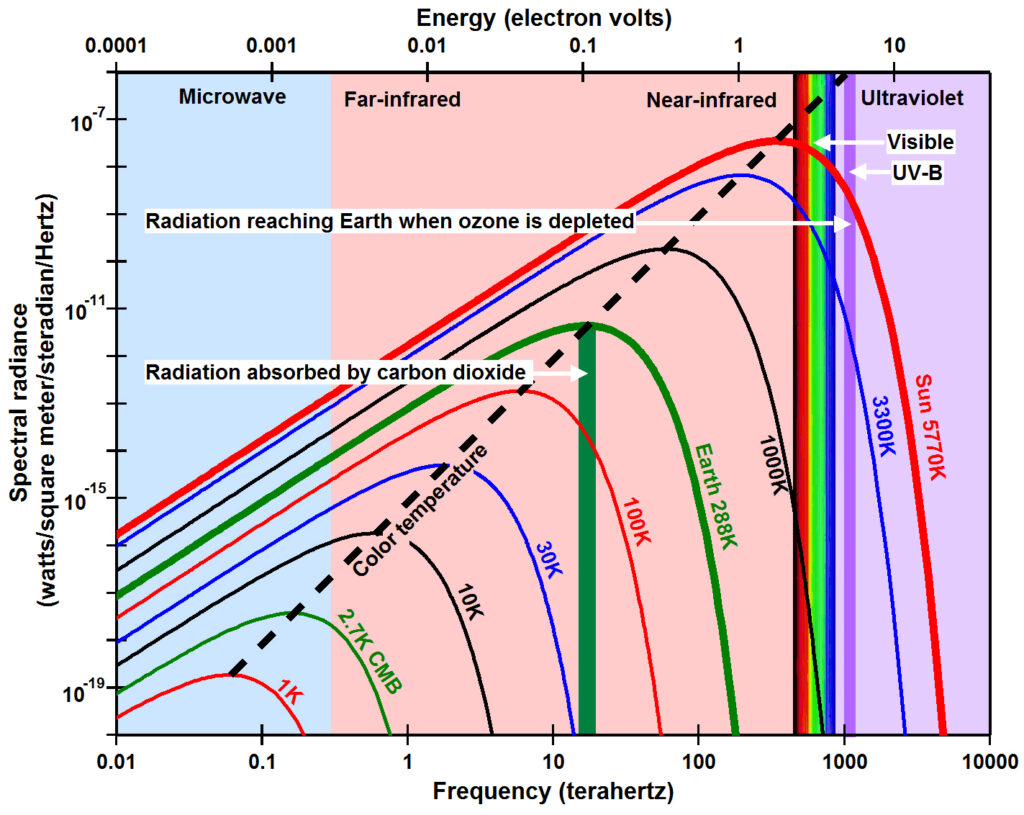# The Primary Problem with Greenhouse Warming Theory

This website describes a wealth of evidence showing that ozone depletion theory explains the location, timing, and amount of global warming observed recently and throughout geologic history far more directly and clearly than greenhouse gas theory. This sounds like heresy at a time when the vast majority of climate scientists are convinced beyond a reasonable doubt that greenhouse gas theory explains global warming quite precisely. Given that climate science is under withering attack primarily by non-scientists viewed as being motivated more by fear of financial loss than by a desire to understand what is actually happening, heretical-sounding science is an anathema. The unfortunate result of this stalemate has been to inhibit scientific debate. While consensus is the stuff of politics, debate is the stuff of science. And the greatest strength of science is that it is ultimately self-correcting in light of new and improved evidence.The energy of radiation absorbed by carbon dioxide around 14,900 nanometers (blue circle) is near 0.08 electron volts (green circle) while the energy that reaches Earth when the ozone layer is depleted around 310 nanometers (red circle) is near 4 electron volts, 48 times larger.

The primary problem with greenhouse gas theory is that it is based on the pervasive assumption that electromagnetic radiation propagates through space and through Earth’s atmosphere in a manner similar to waves in matter so that radiant energy is proportional to the square of the amplitude of the waves and is thus summable across some finite bandwidth. From this perspective, the total infrared energy absorbed by carbon dioxide over the broad band of wavelengths from 13,100 to 17,300 nanometers (blue circle in the figure to the right) is much larger than the total ultraviolet-B energy that reaches Earth when ozone is depleted in the very narrow wavelength band from 300 to 330 nanometers (red circle).

But there is no matter in space. Electromagnetic radiation in space is an electromagnetic field where energy is equal to frequency times a constant as postulated by Max Planck in 1900. From this perspective, infrared energy absorbed by carbon dioxide is in the range of 0.08 electron volts (green circle) while ultraviolet-B radiation has energy around 4 electron volts (red circle), 48 times larger.Planck’s law for the spectral radiance (B) as a function of frequency cubed (ν3) emitted by a black body at temperature T.

(Planck, 1900) derived empirically an equation for the spectral radiance (the amount of radiance at each frequency) radiated by a black body as a function of frequency cubed (ν3, the Greek letter nu) and as a function of the absolute temperature (T) of the surface of that body. Planck’s equation combines the success of Wien’s approximation in predicting spectral radiance at high frequencies with the success of the Rayleigh-Jeans law, formally defined in 1905, in predicting spectral radiance at low frequencies.

To derive his law, Planck had to postulate that the energy (E) contained in radiation equals the frequency of the radiation (ν) times a constant (h) that has become known as the Planck constantE=hν shows up in Planck’s law in the numerator of the exponent of e, the base of the natural logarithm, where the denominator of the exponent (kBT) is the Boltzmann constant (kB) times temperature (T), the thermal energy carried by each microscopic degree of freedom within the system.

E=hν is the energy that must be added to a physical/chemical system, typically through radiation, in order to cause a chemical reaction. In photochemistry,  is used in chemical equations to signify the energy absorbed that causes photoionization or photodissociation. For example, frequency must be greater than 3000 terahertz to ionize nitrogen (N2) or greater than 1237 terahertz to dissociate oxygen (O2). Infrared radiation, frequency less than 430 terahertz, does not have enough energy to penetrate glass. Visible light, frequencies between 430 and 790 terahertz, has enough energy to cause photosynthesisUltraviolet radiation, frequencies greater than 790 terahertz, begins to damage DNA, causing sunburn and skin cancerX-rays with frequencies greater than 30,000 terahertz have enough energy to penetrate your body but will also destroy your body unless the amount, the dosage, is minimal. Note the distinct difference between the very high frequencies of X-rays versus the amount or dosage describing how much of this particular frequency exists or the duration of exposure to this amount of radiation.

Frequency, the color of radiation, does not change over galactic distances except for Doppler effects, while amount (brightness, intensity, flux) decreases inversely with the square of the distance as the radiation spreads out from its source. This complete separation between frequency and amount for radiation is distinctly different from waves in matter. The energy of waves in matter deforms the bonds holding matter together attenuating both frequency and amplitude with distance. Radiation is nothing more than frequency transmitted by oscillations of the bonds that hold matter together, just as oscillations in a radio station’s antenna transmits the frequency assigned to the radio station. Radiation is simply thermal energy transmitted through space and air. Radiation is absorbed in matter.

This separation of frequency and amount is shown clearly by the photoelectric effect, the observation that when certain frequencies of light shine on certain metals, electrons are emitted, but only when the frequency of light equals or exceeds some threshold frequency. Below that frequency no electrons can be emitted no matter the amount of light nor the duration of the exposure. Einstein (1905) (A version in English), building on Planck’s E=hν, explained the photoelectric effect in terms of light quanta, leading to the concept of the photon (Lewis, 1926) and modern quantum electrodynamics.A prism divides white light into its components. The total energy of the white light is not equal to the sum of the energies of each color in this rainbow as currently assumed when calculating the thermal effects of greenhouse gases.

Since energy in space is simply frequency, the energy at different frequencies cannot be added together. Radiation that contains red light at 1.8 electron volts (eV), yellow light at 2.1 eV, green light at 2.3 eV, blue light at 2.6 eV, and violet light at 3.0 eV does not contain a total energy of 11.8 eV, the energy of extreme ultraviolet radiation only found in the uppermost parts of Earth’s atmosphere. It just contains some energy at each color.

While Planck was convinced that energy in radiation is equal to frequency times a constant, he also thought that the spectral radiance measured was energy, as it would be for waves in matter. In fact he ultimately derived his law assuming a finite number of mechanical oscillators in a cavity. But if E=hν, then energy should be plotted along the x-axis as shown at the top of the figure (below left) and the Planck constant h should not be included as a primary factor in Planck’s law because it has units of electron volts per cycle per second or joules per cycle per second. Energy is frequency (x-axis) and spectral radiance (y-axis) should be amount. To reiterate, frequency and amount are distinctly different for electromagnetic radiation in space, but intermixed for waves in matter.Energy is a function of frequency and should therefore be plotted on the x-axis (top of this figure) and units of watts should not be included on the y-axis. The colored lines show the spectral radiance predicted by Planck’s law for black bodies with different absolute temperatures.

This primary problem with greenhouse-gas theory, the question of whether light is a wave or a particle, has been ubiquitous throughout the history of physics and is still not clearly resolved. In Classical GreeceAristotle proposed that light was a disturbance in the element air, a wavelike phenomenon, while Democritus thought light was some form of solar atom, particle-like. By 1630, René Descartes explained observations of light by modeling wave-like disturbances in some medium, but Isaac Newton thought of light as corpuscularRobert HookeChristiaan Huygens, and Agustin-Jean Fresnel developed the mathematics for light as waves. Young’s double-slit experiment in 1801 convinced many physicists that light must be wavelike, but developments in chemistry ultimately leading to the discovery of the atom and the periodic table kept the particle nature of light alive.

In 1865, James Clerk Maxwell proposed a theory of the electromagnetic field that occupies “the space in the neighborhood of the electric and magnetic bodies” and “assumes that in that space there is matter in motion, by which the observed electromagnetic phenomena are produced” (Maxwell, 1865)Maxwell’s equations are widely used today, forming the foundation of classical electrodynamics, classical optics, and electric circuits and they are the reason we all think of radiation in terms of waves in something. This led to the search for the luminiferous aether, the medium in which light must propagate. This search was a major effort of many physicists until the Michelson-Morley experiment in 1887 could not detect the aether and Einstein’s special relativity provided an alternative.

In modern physics, electromagnetic radiation is understood through the principle of wave particle duality, the concept that “all matter exhibits the properties of not only particles, which have mass, but also waves, which transfer energy” (Wikipedia). But electromagnetic radiation in space is not matter as usually defined as an assemblage of atoms and molecules, and there is no matter in space through which waves can propagate. Therefore, I propose that we should think in terms of field-particle-wave triality or vacuum-gas-solid triality as explained on the web page .